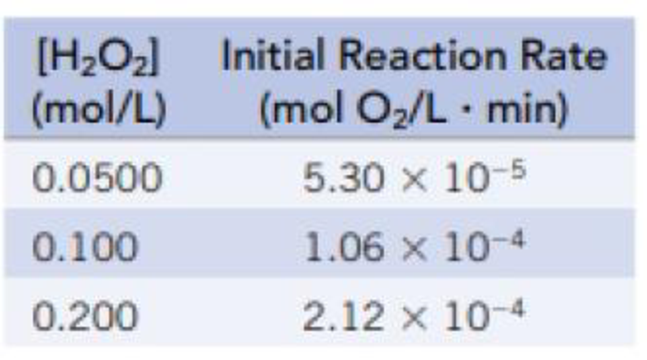# Hydrogen peroxide, H 2 O 2 (aq), decomposes to H 2 O( ℓ ) and O 2 (g) 2 H 2 O 2 (aq) → 2 H 2 O( ℓ ) + O 2 (g) At a particular temperature, the following data were collected for the initial rate of appearance O 2 . (a) What is the rate law for this reaction? (b) Calculate the value of the rate constant for this reaction. (c) If the chemical equation for this reaction is written as H 2 O 2 (aq) → H 2 O( ℓ ) + ½ O 2 (g) what is the value of the rate constant?### Chemistry & Chemical Reactivity

9th Edition
John C. Kotz + 3 others
Publisher: Cengage Learning
ISBN: 9781133949640

#### Solutions

Chapter
Section### Chemistry & Chemical Reactivity

9th Edition
John C. Kotz + 3 others
Publisher: Cengage Learning
ISBN: 9781133949640
Chapter 14, Problem 74GQ
Textbook Problem
10 views

## Hydrogen peroxide, H2O2(aq), decomposes to H2O(ℓ) and O2(g)2 H2O2(aq) → 2 H2O(ℓ) + O2(g)At a particular temperature, the following data were collected for the initial rate of appearance O2.(a) What is the rate law for this reaction?(b) Calculate the value of the rate constant for this reaction.(c) If the chemical equation for this reaction is written asH2O2(aq) → H2O(ℓ) + ½ O2(g)what is the value of the rate constant?

(a)

Interpretation Introduction

Interpretation:

The rate law has to be determined for the given reaction.

Concept introduction:

Rate law: It is an equation that related to the dependence of the reaction rate on the concentration of each substrate (reactants).

For a reaction,

aA+bB+cCProducts

Where,

A and B are reactants

a and b are stoichiometric coefficients

Rate=-Δ[A]Δt=k[A]x[B]y[C]z

Where,

k is the rate constant

Rate constant: Rate constant is an expression used to relate the rate of a reaction to the concentration of reactants participating in the reaction.

### Explanation of Solution

Given information,

The reaction is 2H2O2(aq)2H2O(l)+O2(aq)

[H2O2](mol/L)InitialReactionRate(molO2/L.min)0.05005.30×1050.1001.06×1040.2002

(b)

Interpretation Introduction

Interpretation:

The value of rate has to be determined for the given reaction.

Concept introduction:

Rate law: It is an equation that related to the dependence of the reaction rate on the concentration of each substrate (reactants).

For a reaction,

aA+bB+cCProducts

Where,

A and B are reactants

a and b are stoichiometric coefficients

Rate=-Δ[A]Δt=k[A]x[B]y[C]z

Where,

k is the rate constant

Rate constant: Rate constant is an expression used to relate the rate of a reaction to the concentration of reactants participating in the reaction.

(c)

Interpretation Introduction

Interpretation:

The value of rate has to be determined for the given reaction.

Concept introduction:

Rate law: It is an equation that related to the dependence of the reaction rate on the concentration of each substrate (reactants).

For a reaction,

aA+bB+cCProducts

Where,

A and B are reactants

a and b are stoichiometric coefficients

Rate=-Δ[A]Δt=k[A]x[B]y[C]z

Where,

k is the rate constant

Rate constant: Rate constant is an expression used to relate the rate of a reaction to the concentration of reactants participating in the reaction.

Rate constant for a particular reaction is always constant. It does not depend on the concentration of the reactant.

### Still sussing out bartleby?

Check out a sample textbook solution.

See a sample solution

#### The Solution to Your Study Problems

Bartleby provides explanations to thousands of textbook problems written by our experts, many with advanced degrees!

Get Started

Find more solutions based on key concepts
Identify two critical periods of life that demand high nutrient intakes, and defend the use of a vegetarian die...

Nutrition: Concepts and Controversies - Standalone book (MindTap Course List)

Describe how bone first develops.

Human Biology (MindTap Course List)

What kind of spectrum does a neon sign produce?

Horizons: Exploring the Universe (MindTap Course List)

Use energy diagrams to compare catalyzed and uncatalyzed reactions.

Chemistry for Today: General, Organic, and Biochemistry

Name each of the compounds a. Au2O b. NiO c. SnO2 d. SnO

General, Organic, and Biological Chemistry

In the Bohr model of the hydrogen atom, an electron moves in a circular path around a proton. The speed of the ...

Physics for Scientists and Engineers, Technology Update (No access codes included)

What are algae? Are all algae seaweeds?

Oceanography: An Invitation To Marine Science, Loose-leaf Versin